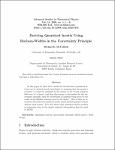## Deriving quantised inertia using horizon-widths in the uncertainty principle2020
McCulloch, M. E.
##### Abstract
In this paper we show that a model for inertial mass (quantised inertia) can be derived exactly and simply by assuming that the property of inertia is caused by gradients in the energy in the Unruh radiation field seen by a object, and that this energy is determined by the uncertainty principle, with the uncertainty in position being given by the width of the Rindler horizons seen by the object. This is then an alternative derivation for quantised inertia which predicts galaxy rotation without dark matter. It is also shown that quantised inertia predicts an expression close to the simple empirical interpolation function used in MoND.
##### Journal or Serie
Advanced Studies in Theoretical Physics, 2020, vol. 14, núm. 1, p. 1-8